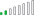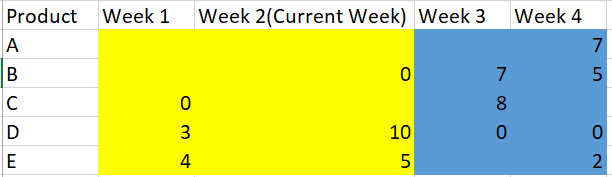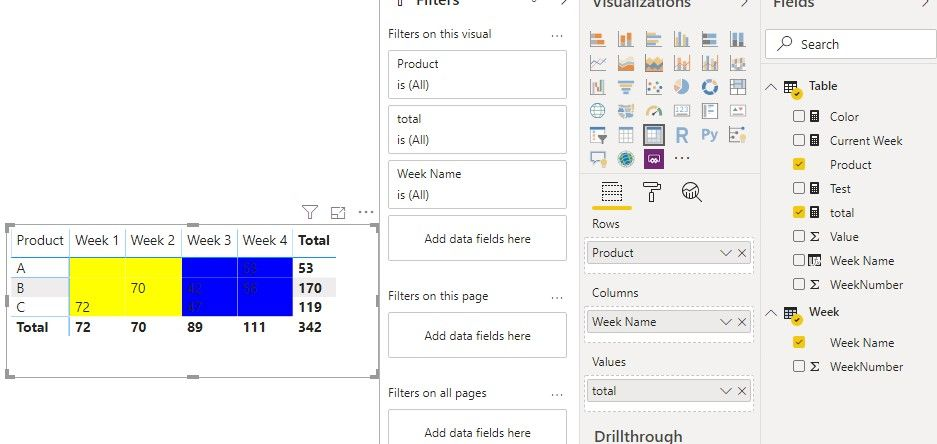cancel
Showing results for
Did you mean:Resolver II

## Color Code Blank value

Hi All,

I am facing an issue while applying conditional formatting. Please find below requirement with expected output and current output.

Note: 1) The values are sum of sales.

2) Blank means product was not available for a particular week hence blank

3) Blank can't be replaced with 0. That's the business requirement

Expected : The requirement is when week number<= current week, then color code yellow else blue.But instead of the above, I get this:Issue: The DAX is not taking blank values into consideration. I have applied 3-4 methods. But none of them are working on blank values.

I have tried:  if( week number<= current week && Sum(Sales)) = BLANK(), then color code yellow else blue.

Can anybody please let me know the solution of this problem?

Thanks,

Himanshu

1 ACCEPTED SOLUTIONCommunity Support

Hi @himanshu56 ,

We can create a measure as value field after create a spreated calculated table as the column header:

Calculated table:

``Week = ADDCOLUMNS(DISTINCT('Table'[WeekNumber]),"Week Name","Week "&[WeekNumber])``Measure:

``total = IF(COUNTROWS('Table')=0,BLANK(),CALCULATE(SUM('Table'[Value]),FILTER('Table','Table'[WeekNumber] in DISTINCT('Week'[WeekNumber])))) & ""``

Color Field Measure:

``Color = IF(SELECTEDVALUE('Week'[WeekNumber])<=[Current Week],"#FFFF00","#0000FF")``Best regards,

Community Support Team _ Dong Li
If this post helps, then please consider Accept it as the solution to help the other members find it more quickly.
2 REPLIES 2Community Support

Hi @himanshu56 ,

Best regards,

Community Support Team _ Dong Li
If this post helps, then please consider Accept it as the solution to help the other members find it more quickly.Community Support

Hi @himanshu56 ,

We can create a measure as value field after create a spreated calculated table as the column header:

Calculated table:

``Week = ADDCOLUMNS(DISTINCT('Table'[WeekNumber]),"Week Name","Week "&[WeekNumber])``Measure:

``total = IF(COUNTROWS('Table')=0,BLANK(),CALCULATE(SUM('Table'[Value]),FILTER('Table','Table'[WeekNumber] in DISTINCT('Week'[WeekNumber])))) & ""``

Color Field Measure:

``Color = IF(SELECTEDVALUE('Week'[WeekNumber])<=[Current Week],"#FFFF00","#0000FF")``Best regards,

Community Support Team _ Dong Li
If this post helps, then please consider Accept it as the solution to help the other members find it more quickly.